# Shear Stress Calculator

Created by Luis Fernando
Reviewed by Steven Wooding
Last updated: May 24, 2022

This shear stress calculator calculates the horizontal shear stress due to transverse loads and the shear stress owed to torsion applied on a circular shaft.

The shear stress owed to transverse forces is critical in the design of thin-walled members. For non-tin-walled members, like a circular shaft, the most important will probably be the axial stress caused by the bending moment, and you'll likely need to use our section modulus calculator. Our stress calculator can also be helpful if you're dealing with axial stress. If that's not the case, use this calculator!

The shear stress equation to use will depend on whether we apply a transverse load to a beam or a torsion couple to a circular shaft. In the next section, you can look at the under these two conditions.

Shear stress is not only present in solid bodies; it's also an essential quantity in the study of liquids and gases. For this reason, we created a dedicated to answering questions like how to calculate the shear stress of a fluid or how to calculate its viscosity from shear stress and shear rate.

## Shear stress formula for transverse shear

Stress is defined as force per unit area. In a beam section subjected to shear force, the average shear stress in that section is:

$\tau_{\text{avg}} = \frac{V}{A}$

This is just an average, as the shear stress across a beam subjected to transverse shear varies widely.

You can find the exact shear stress at any distance from the centroidal axis with the following equation:

$\tau = \frac{VQ}{It}$

where:

• $\tau$ – Shear stress at a specific distance from the neutral axis;
• $V$ – Internal shear force at the section of interest in the beam;
• $Q$ – First moment, about the neutral axis, of the cross-sectional area that lies above the point where we'll calculate the stress;
• $t$ – Section width at the point where we'll calculate the shear stress; and
• $I$ – Second moment of area of the entire beam cross-section, about the neutral axis (you can find it with our moment of inertia calculator).

The previous equation also gives us an average value of the stress, as it doesn't only vary with vertical distance from the neutral axis but also across the width of the beam. Fortunately, this variation is usually minor, and we can neglect it.

## How to calculate shear stress from torque

To calculate the shear stress from a torque applied to a circular shaft, use the torsional shear stress formula:

$\tau = \frac{T \rho}{J}$

where:

• $\tau$ – Shear stress at the point of interest;
• $\rho$ – Radial distance from the shaft center to the point where we want to calculate the stress;
• $T$ – Torque applied to the shaft; and
• $J$Polar moment of inertia of inertia of the cross-section.
• For a solid shaft of radius c, $J = π c^4/2$.
• For a hollow shaft of inner radius $c_1$ and outer radius $c_2$, $J = π(c_2^4-c_1^4)/2$.

## How to calculate maximum shear stress from torque

The maximum shear stress for torsional loads occurs at the outer surface of the circular shaft, where $\rho = c$ or $\rho = c_2$. In other words, the maximum shear stress is given by:

$\tau_{max} = \frac{Tc}{J}$

where:

• $\tau_{max}$ — Maximum shear stress; and
• $c$ — Shaft radius.

You must also consider this when using these formulas:

• The shear stress units are psi or pascals.
• This formula only applies to homogenous materials with a linear elastic behavior.

## Formulas to calculate maximum shear stress under transverse shear load.

As mentioned before, the maximum shear stress of a circular beam under torsion is the shear stress at its outermost part. But if you're interested in how to calculate the maximum shear stress for a beam under transverse shear $V$, it's not that easy.

Fortunately, our calculator can do it for you using the following formulas for different beams under transverse shear $V$:

Form

Maximum shear stress

Rectangle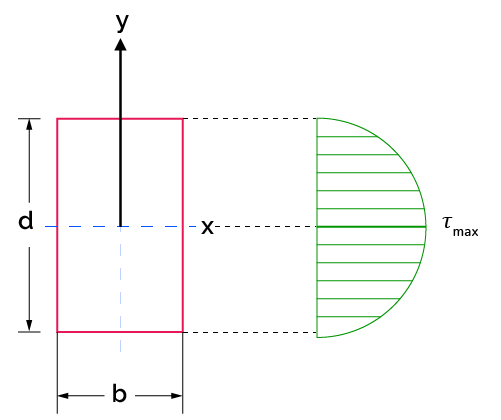$\tau_{max} = \frac{3V}{2A}$

$A = db$

Stress at distance y:

$\tau = \frac{3V}{2A}(1-\frac{y^2}{(d/2)^2})$

Hollow circle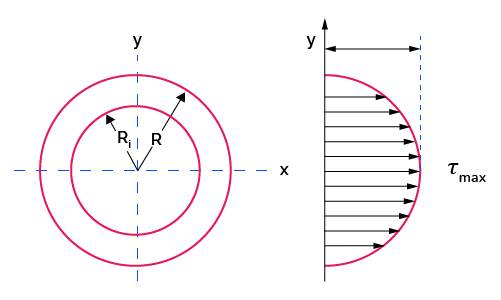$\tau_{max} = \frac{4V}{3A}\frac{R^2+RR_i+{R_i}^2}{R^2+{R_i}^2}$

I-beam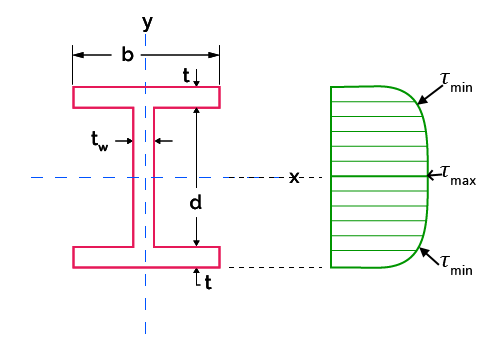$\tau_{max} = \frac{V}{8\ I_xt_w} \left(b(d+2t)^2 - bd^2+t_wd^2\right)$

$\tau_{min} = \frac{V}{8\ I_xt_w} \left((d+2t)^2 - d^2\right)$

$I_x = \frac{b(d+2t)^3-(b-t_w)d^3}{12}$

In an I-beam (wide-flange beam), the $\tau_{min}$ value (the stress value at the joint between the flange and web) is a critical quantity, as sometimes the joint in that zone is created through welding or glue, and we need to calculate how much stress those elements will withstand.

## Important considerations when using these shear stress equations and the calculator

• When we apply a transverse load to a beam, transverse and longitudinal shear stresses arise. Due to the complementary property of shear, these longitudinal and transversal stresses have the same magnitude. Therefore, the horizontal shear stress calculation only requires knowing the transverse shear stress value, as they are both the same.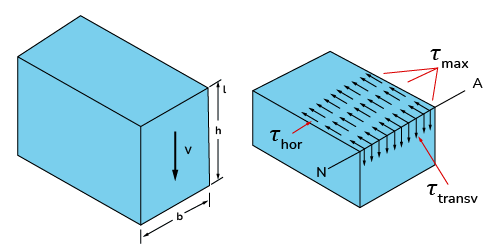Rectangular beam under transverse shear VVV. τmax\tau_{max}τmax​ occurs at the horizontal centroidal axis, where QQQ is maximum.
• $Q$ is a tricky quantity. Mathematically, it is the area above the point of interest multiplied by the distance between the centroid of that area to the neutral axis. For example, for a cross-section with cam geometry, if you wanted to calculate the shear stress at a distance $y'$ from the neutral axis, the corresponding $Q$ would be $Q = \bar y' \bar A$.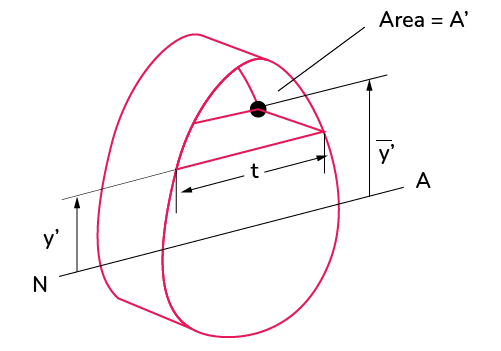• From the previous image, we can also note what the $t$ term means.

## What are the shear stress units?

The shear stress units are pascal (Pa) in the International System of Units and pound per square inch (psi) in the United States customary units system. We can also describe this quantity in bar units.

## FAQ

### What is the polar moment of inertia of a circle of 10 cm radius?

The polar moment of inertia of a circle of 10 cm radius is 15708 cm4. For the United States customary units system, its polar moment of inertia is 377.4 in4.

### How do I calculate the shear stress of a fluid?

To calculate the shear stress 𝜏ᵧₓ of a fluid:

1. Determine whether it is a Newtonian or non-Newtonian fluid.
2. For a Newtonian fluid, multiply the viscosity μ by the shear rate du/dy: 𝜏ᵧₓ = μ(du/dy).
3. If it's non-Newtonian, multiply the apparent viscosity η by the shear rate du/dy:
𝜏ᵧₓ = η(du/dy).

η and μ are analog terms, with the difference that η varies with du/dy.

### How do I calculate viscosity from shear stress and shear rate?

To calculate viscosity μ from shear stress 𝜏ᵧₓ and shear rate du/dy, divide the shear stress by the shear strain:

μ = 𝜏ᵧₓ/(du/dy)

### How do I calculate shear stress from flow rate?

To calculate shear stress from the flow rate in a pipe, use the formula 𝜏ᵣₓ = -(4μrQ)/(πR⁴), where:

• 𝜏ᵣₓ – Shear stress (in the flow direction) at the point of interest;
• μ – Absolute (or dynamic) viscosity of the fluid;
• r – Radial distance from the pipe center to the point of interest;
• Q – Flow rate; and
• R – Pipe radius.

That's how you calculate shear stress from flow rate! Take into account that this formula applies to Newtonian fluids under fully developed laminar flow.

### How do I calculate shear stress in bolts?

The maximum shear stress of a screw body subjected to torsion can be expressed as:

𝜏ₘₐₓ = 16T / (πdᵣ³)

where:

• 𝜏ₘₐₓ – Maximum nominal shear stress;
• T – Torque applied to the screw; and
• dᵣ – Minor diameter of the screw.
Luis Fernando
Inputs
Select a shape
Shear stress in a beam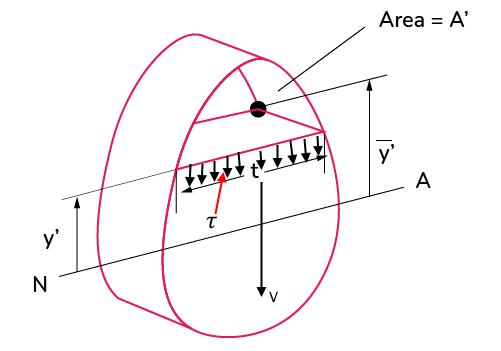Calculate the shear stress in an arbitrary beam subjected to a shear force V, using the shear formula 𝜏 = VQ/It.
Additionally, you can use the section modulus calculator to find the centroid and area moment of inertia of many structural shapes, which will help you to find Q, I and t.
Shear force magnitude (V)
N
Width (t)
m
First moment of area (Q = ȳ'A')
Moment of inertia (I)
m⁴
Shear stress magnitude (𝜏)
Pa
People also viewed…

### Coefficient of discharge

Estimate the ratio between theoretical and actual discharge values using the coefficient of discharge calculator.

### Flat vs. round Earth

Omni's not-flat Earth calculator helps you perform three experiments that prove the world is round.

### Gravitational time dilation

With this gravitational time dilation calculator you can check how much time slows down by near big objects.